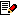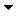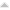vectorMath, crossProduct, scalarProductFrom: bemfarmer 21 Feb 2012  (1 of 8)Michael, does the following code appear ok for manipulation of vectors in MoI? Is there a better way? In the script that is being created, I'm using your dot product code, and length and normalize from your bisector script. Needed is cross product of 2 vectors, and also scalar product. // Michael's MoI bisector script has the needed code for dot product, length, and // normalize. Reference book: Geometric Tools for Computer Graphics. Section 4.4 // of the book, page 117, shows the dot product code. Page 119 shows the cross // product code, which is similarly converted to MoI type javascript, using v0 // instead of the u1 vector used in the book. Note that v0 and v1 are Vectors, // and v0.x, v0.y, v0.z, v1.x, v1.y, and v1.z, are the x, y, and z parts of the vectors. // (crossproduct creates the new "pseudo" vector, which is still a vector.) // Scalar formula is on page 115. // Elsewhere I've read that: "The individual components of a 3D vector can be accessed through its x, y and z member variables." function crossProduct( v0, v1, startpt ) { var vcx = (v0.y * v1.z) - (v0.z * v1.y); var vcy = (v0.z * v1.x) - (v0.x * v1.z); var vcz = (v0.x * v1.y) - (v0.y * v1.x); var vcross_endpt = moi.vectorMath.createPoint( vcx, vcy, vcz ); var vcross = moi.vectorMath.makeVector( startpt, vcross_endpt); return vcross; } function scalarProduct( v0, a, startpt ) { var vcx = v0.x * a; var vcy = v0.y * a; var vcz = v0.z * a; var vscalar-endpt = moi.vectorMath.createPoint( vcx, vcy, vcz ); var vscalar = moi.vectorMath.makeVector( startpt, vscalar-endpt); return vscalar; } vectorMath.makeVector is not in the documentation. Are there any other undocumented vectorMath methods? The difference between Points and Vectors, and their display, is confusing to me. I'm thinking Points are displayed as the endpoints of vectors, from some origin, and vectors are displayed as start and end points... Thank you. EDITED: 21 Feb 2012 by BEMFARMERReply MoreFrom: Michael Gibson 21 Feb 2012  (2 of 8)4951.2 In reply to 4951.1 Hi Brian, > vectorMath.makeVector is not in the documentation. > Are there any other undocumented vectorMath methods? Which documentation are you looking at? The only thing that I've really put out myself is the moi.idl file which you can get from this page here: http://moi3d.com/wiki/Scripting That does list makeVector in it, look for the "interface IMoiVectorMath" section to see what methods moi.vectorMath has available in it. makeVector is just a convenience function that takes 2 points and returns a vector between the 2 points by subtracting them. So if you do makeVector( ptA, ptB ) where ptA and ptB are some points, the return will be ptB - ptA which is a directional vector going from ptA to ptB. I'll take a look at your code here in a minute. - MichaelReply MoreFrom: Michael Gibson 21 Feb 2012  (3 of 8)4951.3 In reply to 4951.1 Hi Brian, > The difference between Points and Vectors, and their > display, is confusing to me. > I'm thinking Points are displayed as the endpoints of > vectors, from some origin, and vectors are displayed as > start and end points... Well, both points and vectors are represented by the same data - a triplet of x,y,z data. The difference is the way that data is interpreted - for a point the x,y,z is meant to indicate a specific location in space. For a vector the x,y,z is meant to represent a direction rather than a position. Search for something like "vector algebra" to see examples, like: http://emweb.unl.edu/math/mathweb/vectors/vectors.html - MichaelReply MoreFrom: Michael Gibson 21 Feb 2012  (4 of 8)4951.4 In reply to 4951.1 Hi Brian, Your code was: quote: function crossProduct( v0, v1, startpt ) { var vcx = (v0.y * v1.z) - (v0.z * v1.y); var vcy = (v0.z * v1.x) - (v0.x * v1.z); var vcz = (v0.x * v1.y) - (v0.y * v1.x); var vcross_endpt = moi.vectorMath.createPoint( vcx, vcy, vcz ); var vcross = moi.vectorMath.makeVector( startpt, vcross_endpt); return vcross; } So this is a kind of weird way to do cross product - normally the cross product takes in 2 vectors as input and returns one new vector as output which is perpendicular to both inputs, unless they were colinear in which case it will be zero magnitude vector (or almost zero magnitude due to the tiny bit of fuzz in floating point calculations). The part that you may be getting stuck on is that to build a vector directly from x, y, z values you actually want to use createPoint() to do that, since you will actually hold a point or a vector in the exact same point object since they both just need to contain an x,y,z triplet value. Since both vectors and points have the exact same data in them, there's just one class for them since otherwise they would just have all the exact same properties and methods and everything. So the return value from crossProduct should be sent back with createPoint() - you only use makeVector for building a vector that represents a displacement between a "from point" and a "to point". So a more normal cross product function would look like this: code:```function crossProduct( v0, v1 ) { var x = (v0.y * v1.z) - (v0.z * v1.y); var y = (v0.z * v1.x) - (v0.x * v1.z); var z = (v0.x * v1.y) - (v0.y * v1.x); return moi.vectorMath.createPoint( x, y, z ); } ``` And normally the result of a scalar product/dot product does not produce a vector as output, but instead generates a single number as the output. It's not the same thing as "scaling" a vector. So normally the dot product would be like this: code:```function dotProduct( v0, v1 ) { return (v0.x * v1.x) + (v0.y * v1.y) + (v0.z * v1.z); } ``` If you want to scale up a vector to increase or decrease its magnitude you would do something like this: code:```function scaleVector( v, factor ) { var x = v.x * factor; var y = v.y * factor; var z = v.z * factor; return moi.vectorMath.createPoint( x, y, z ); } ``` That one produces a new scaled vector as output from the function. There is also a method .scale( ) that you can call on a point or vector to modify that vector by a scale factor. To do that you would do something like this: var vector = vector.scale( 5.0 ); That .scale method is on IMoiPoint - look up IMoiPoint in the moi.idl file to see what else is there - that's also where you will see the properties for x, y, z listed. There's not a whole lot of stuff set up for scripting this stuff yet - in the future I want to expand the library of functions available to script for doing this kind of stuff. Right now you will need to make some various helper functions of your own though. Hope this helps! - Michael EDITED: 21 Feb 2012 by MICHAEL GIBSONReply MoreFrom: bemfarmer 21 Feb 2012  (5 of 8)Thank you very much Michael. Your information is a big help. (The reference book placed such an emphasis on Points and vectors being different...) I should have said "scaling a vector" by multiplying it by a number, not scalar product... I need to have a look at the Moi idl file. I think windows is hiding it... One script coming up, in a week or a month, or ... :-) Edit: (I meant the David Morrill documentation.)Reply MoreFrom: Michael Gibson 21 Feb 2012  (6 of 8)4951.6 In reply to 4951.5 > (The reference book placed such an emphasis on > Points and vectors being different...) It's not necessarily bad to think of them as being different in how they are used, but since they contain the same data pretty often there is just one actual class to represent both of them. - MichaelReply MoreFrom: bemfarmer 21 Feb 2012  (7 of 8)4951.7 In reply to 4951.4 Thank you again Michael. .scale method looks very concise. (My script was making tiny microcurves... )Reply MoreFrom: Frenchy Pilou (PILOU) 21 Feb 2012  (8 of 8)4951.8 In reply to 4951.7 < microcurves no problem a long you don't make micro "black holes" ;) --- Pilou Is beautiful that please without concept! My GalleryReply MoreReply to All